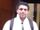## Proc SQL - IF/THEN Conditions

Hi,

I want to do a conditional merge using proc SQL that:

• Searches dataset two within/grouped by ‘ID’ for treatment codes 1 or 2. Something like:By distinct id, IF treatment_code in (1,2) then in_treatment = “Yes” else “No”.
• I want to merge datasets one and two, keeping all values from one and adding the new variable in_treatment that is calculated from two.

data one;

input id name \$;

datalines;

1 Tim

2 Tom

3 Beth

4 Harry

run;

data two;

input id treatment_code;

datalines;

1 1

1 2

2 1

2 4

2 9

3 11

4 2

run;

data want;

id name In_teatment

1   Tim "Yes"

2   Tom``"Yes"

3   Beth "No"

4   Harry "Yes"

;

Thanks! 🙂

6 REPLIES 6

## Re: Proc SQL - IF/THEN Conditions

Data step solution:

``````data one;
input id name \$;
datalines;
1 Tim
2 Tom
3 Beth
4 Harry
;
run;

data two;
input id treatment_code;
datalines;
1 1
1 2
2 1
2 4
2 9
3 11
4 2
;
run;

data two_comp;
set two;
by id;
retain in_treatment '   ';
if first.id then in_treatment = 'No';
if treatment_code in (1,2) then in_treatment = 'Yes';
if last.id then output;
drop treatment_code;
run;

data want;
merge
one
two_comp
;
by id;
run;``````

SQL solution:

``````proc sql;
create table want as
select
a.id,
a.name,
(select
max(
case
when b.treatment_code in (1,2)
then 'Yes'
else 'No'
end
)
from two b
where a.id = b.id
group by b.id
) as in_treatment
from one a
;
quit;``````

Result from both:

```id    name     in_treatment

1    Tim          Yes
2    Tom          Yes
3    Beth         No
4    Harry        Yes
```

Note how much better the code looks when using the "little running man" and {i} buttons for posting.Ksharp
Super User

## Re: Proc SQL - IF/THEN Conditions

``````data one;
input id name \$;
datalines;
1 Tim
2 Tom
3 Beth
4 Harry
;
run;

data two;
input id treatment_code;
datalines;
1 1
1 2
2 1
2 4
2 9
3 11
4 2
;
run;

proc sql;
select a.id,a.name,case when sum(treatment_code in (1,2)) >0 then 'Yes' else 'No ' end as want
from one as a left join two as b
on a.id=b.id
group by a.id,a.name;
quit;``````novinosrin
Tourmaline | Level 20

## Re: Proc SQL - IF/THEN Conditions

``````data one;
input id name \$;
datalines;
1 Tim
2 Tom
3 Beth
4 Harry
;
run;

data two;
input id treatment_code;
datalines;
1 1
1 2
2 1
2 4
2 9
3 11
4 2
;
run;

proc sql;
create table want as
select a.*,ifc(max(treatment_code in (1,2)),'YES','NO') as In_teatment
from one a left join two b
on a.id=b.id
group by a.id,name;
quit;``````novinosrin
Tourmaline | Level 20

## Re: Proc SQL - IF/THEN Conditions

``````data one;
input id name \$;
datalines;
1 Tim
2 Tom
3 Beth
4 Harry
;
run;

data two;
input id treatment_code;
datalines;
1 1
1 2
2 1
2 4
2 9
3 11
4 2
;
run;

data want;
merge one(in=a) two(in=b where=(treatment_code in (1,2)));
by id ;
if a;
if b then In_teatment='YES';
else In_teatment='NO';
if last.id;
run;``````novinosrin
Tourmaline | Level 20

## Re: Proc SQL - IF/THEN Conditions

``````data one;
input id name \$;
datalines;
1 Tim
2 Tom
3 Beth
4 Harry
;
run;

data two;
input id treatment_code;
datalines;
1 1
1 2
2 1
2 4
2 9
3 11
4 2
;
run;

proc sql;
create table want as
select distinct a.*,ifc(treatment_code>.,'YES','NO') as In_teatment
from one a left join two(where=(treatment_code in (1,2))) b
on a.id=b.id;
quit;
``````novinosrin
Tourmaline | Level 20

## Re: Proc SQL - IF/THEN Conditions

``````data one;
input id name \$;
datalines;
1 Tim
2 Tom
3 Beth
4 Harry
;
run;

data two;
input id treatment_code;
datalines;
1 1
1 2
2 1
2 4
2 9
3 11
4 2
;
run;

data want ;
set one;
if _n_=1 then do;
if 0 then set two;
dcl hash H (dataset:'two',multidata:'y') ;
h.definekey  ("id") ;
h.definedata ("treatment_code") ;
h.definedone () ;
end;
length In_teatment \$3;
In_teatment='NO';
do while(h.do_over() eq 0);
if treatment_code in (1,2) then do;
In_teatment='YES';
leave;
end;
end;
run;``````

or

``````data want ;
set one;
if _n_=1 then do;
if 0 then set two;
dcl hash H (dataset:'two(where=(treatment_code in (1,2))',multidata:'y') ;
h.definekey  ("id") ;
h.definedata ("id") ;
h.definedone () ;
end;
length In_teatment \$3;
In_teatment='NO';
if h.check()=0 then In_teatment='YES';
drop treatment_code;
run;``````

Discussion stats
• 6 replies
• 615 views
• 0 likes
• 4 in conversation